We use cookies to enhance your experience on our website. By continuing to use our website, you are agreeing to our use of cookies. You can change your cookie settings at any time. Find out more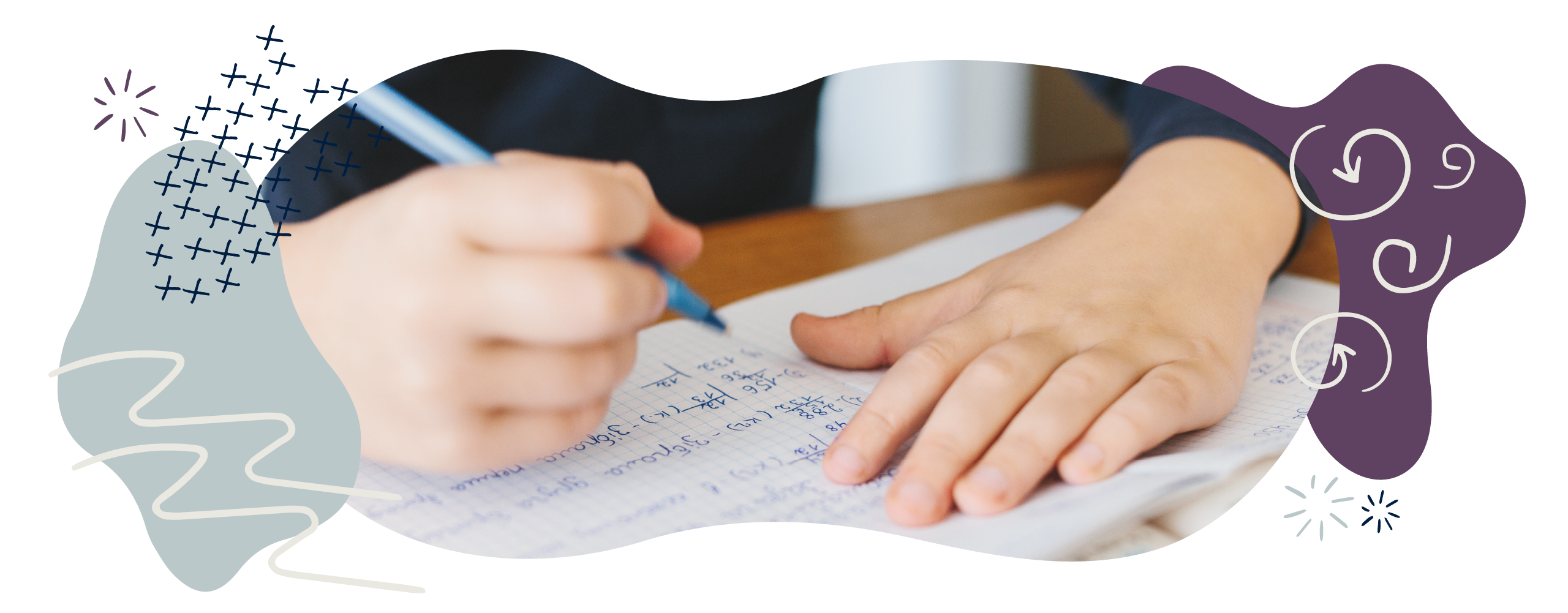# Geometry in Year 5 (age 9–10)

In Year 5, your child will use acute, obtuse, and reflex angles and will draw and measure angles in degrees. They will translate and reflect shapes, and will investigate regular and irregular polygons.

The key words for this section are 2D, 3D, axis, and coordinate.

## What your child will learn

Take a look at the National Curriculum expectations for geometry in Year 5 (ages 9–10):

#### Identify 3D shapes

Your child will recognise and name 3D shapes both in real life and in 2D pictures and drawings.

#### Undertand acute, obtuse, and reflex angles

Your child will know that an angle is a measurement of how much something turns and that angles are measured in degrees.

They should be able to estimate and compare acute (less than 90°), obtuse (between 90° and 180°), and reflex angles (between 180° and 360°).

#### Draw and measure angles in degrees (°)

Your child will draw angles and measure them in degrees (°) using a protractor.

#### Identify angles on a straight line and in turns

Your child will learn that angles around a point add up to 360° and that angles on a straight line add up to 180°.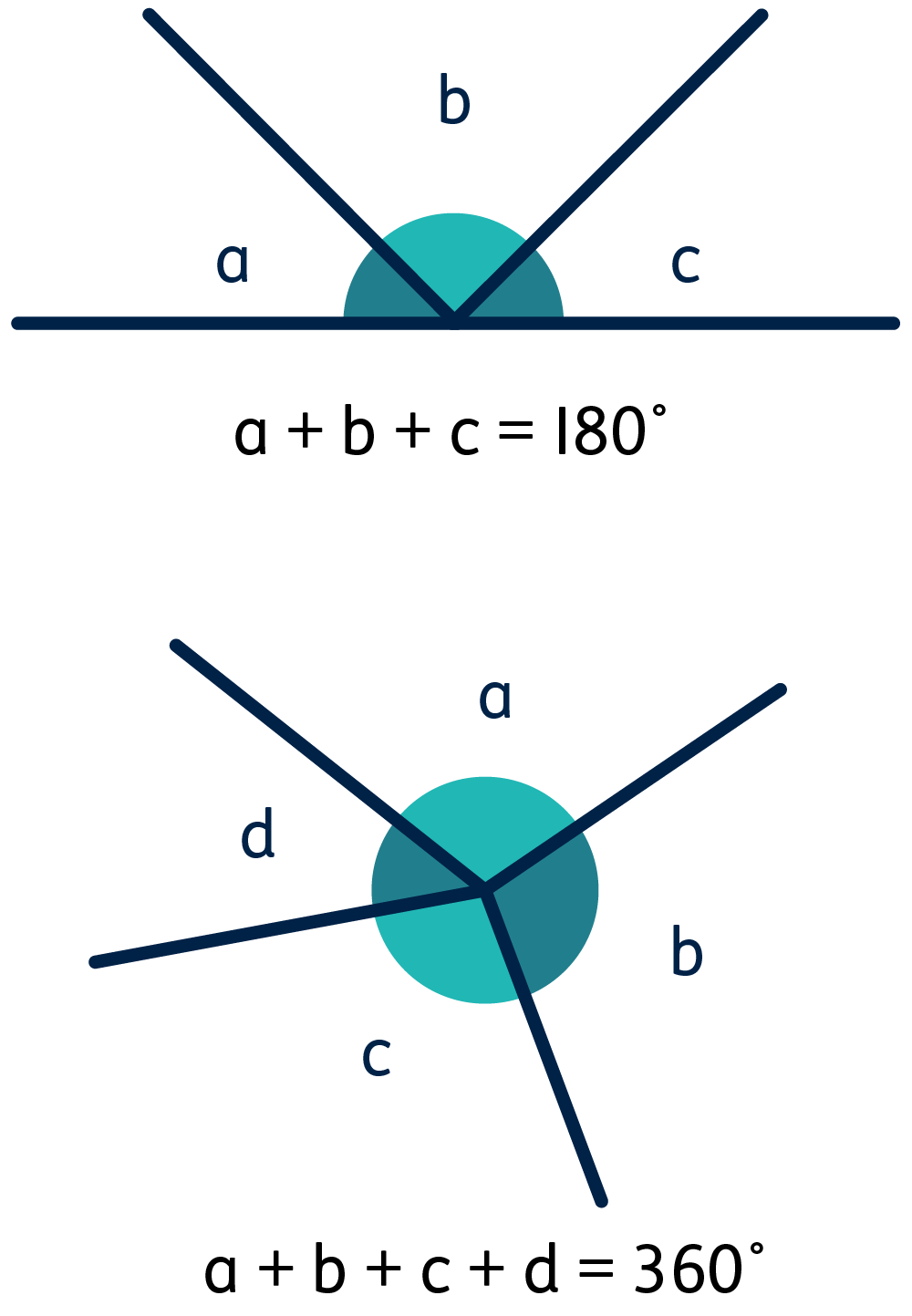They will know that one whole turn measures 360° and a half turn measures 180°. They will also learn to identify multiples of 90°, such as 180°, 270°, 360°, 450°, and so on.

#### Use the properties of rectangles to find missing lengths and angles

Your child will know the properties of rectangles. For instance, they will know that all rectangles have straight sides making up two pairs of parallel lines, four corners, and four right angles, and that the angles in all rectangles add up to 360°.

They should be able to use this knowledge to find missing lengths and angles in rectangles.

#### Distinguish between regular and irregular polygons

Your child will know that a regular polygon is a 2D shape that has equal angles and three or more straight sides of equal length.

They will be able to tell the difference between regular and irregular polygons. They will use language like regular, irregular,polygon, and non-polygon to describe them.

#### Reflect and translate shapes

Your child will be able to show the position of simple shapes on the full coordinate grid using all four quadrants, using positive and negative values for x and y.

They will be expected to describe and record the position of a shape using the full coordinate grid after a shape has been translated or reflected.

## How to help at home

There are lots of everyday ways you can help your child to understand geometry. Here are just a few ideas.

### 1. Use the language of shapes

You can support your child’s learning by helping them to describe shapes in terms of properties, such as:

• The number of sides/edges
• The number of faces
• The number of vertices/corners
• Types of angles within shapes
• Lines of symmetry (if any).

Encourage them to use specific mathematical language such as diagonal, vertical, horizontal, parallel, acute, obtuse, and right angle too.

### 2. Use 3D nets

Have a go at this 3D nets worksheet. See if your child can work out the 3D shapes just from the 2D pictures.

### Activity: Nets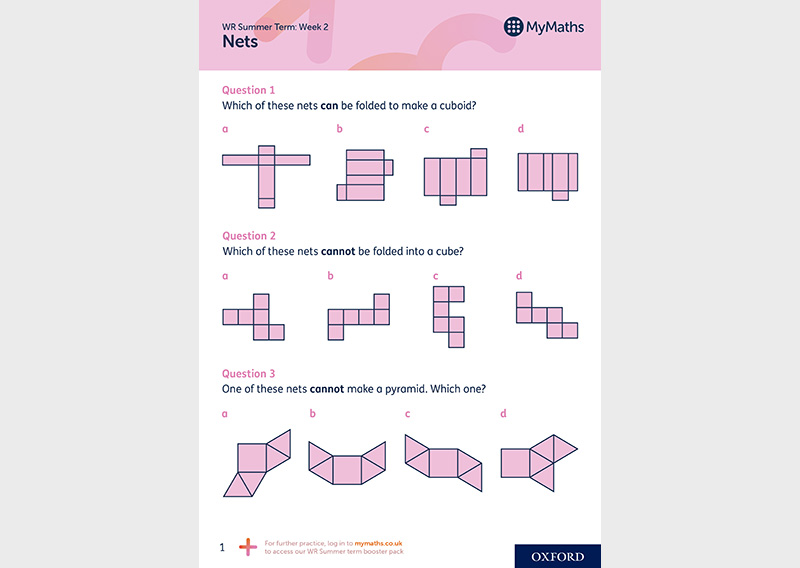Practise making and understanding nets.

### 3. Make a protractor mat

Your child will be expected to draw given angles and measure them in degrees using a protractor.

You can help your child practise using a protractor accurately by making a ‘protractor mat’. Use the corner of a piece of paper to create a 90 degree mat in the shape of a quarter circle. Mark a line for each 10°. Alternatively, create a 180 degree mat in the shape of a half circle. Mark a line for each 10°. This will help them get used to what different increments of degrees look like.

### 4. Find the angles in your name

Your child could write their name (or even just their initials) using straight lines and then measure the angles in their name. Which letters have the most angles? Which letters have the biggest angles?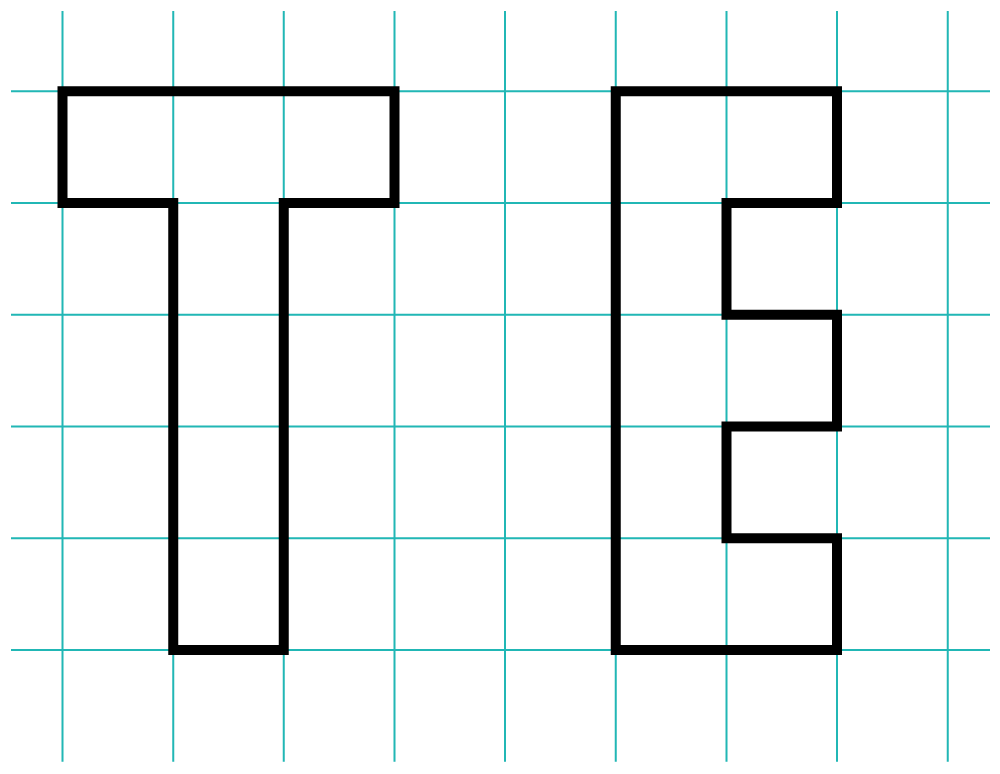### 5. Play Battleships

Coordinates are numbers that determine the position of a point on a map, grid, or graph. Coordinates are recorded using brackets, with the two numbers separated by a comma, like this: (1,2). The first number in a coordinate tells us where the point is on the x-axis (or the horizontal axis). The second number tells us where the point is on the y-axis (or the vertical axis).

You can help your child learn by reading and plotting coordinates. In the example below, the coordinate for the plotted point would be (5,6), where 5 is the value on the x-axis and 6 is the value on the y-axis: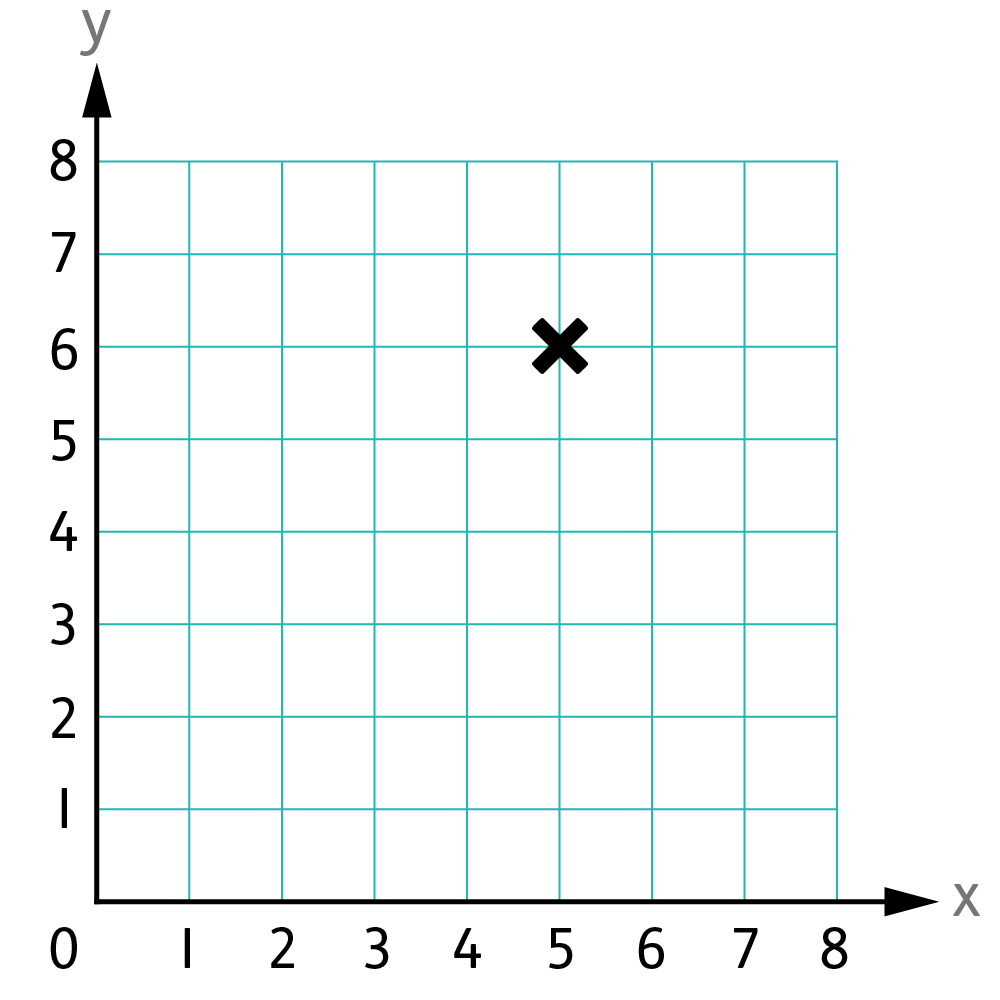Games like Battleships give your child the opportunity to use coordinates. Encourage your child to make their own Battleships grids and play with you or a sibling. This will give them a chance to draw a pair of axes with equal scales and labels, as well as practise finding and describing coordinates.

### 6. Coordinates shape game

Your child will be using coordinates in all four quadrants at school, including the use of negative numbers. Use 1cm-squared paper for your child to draw out a four-quadrant grid like this: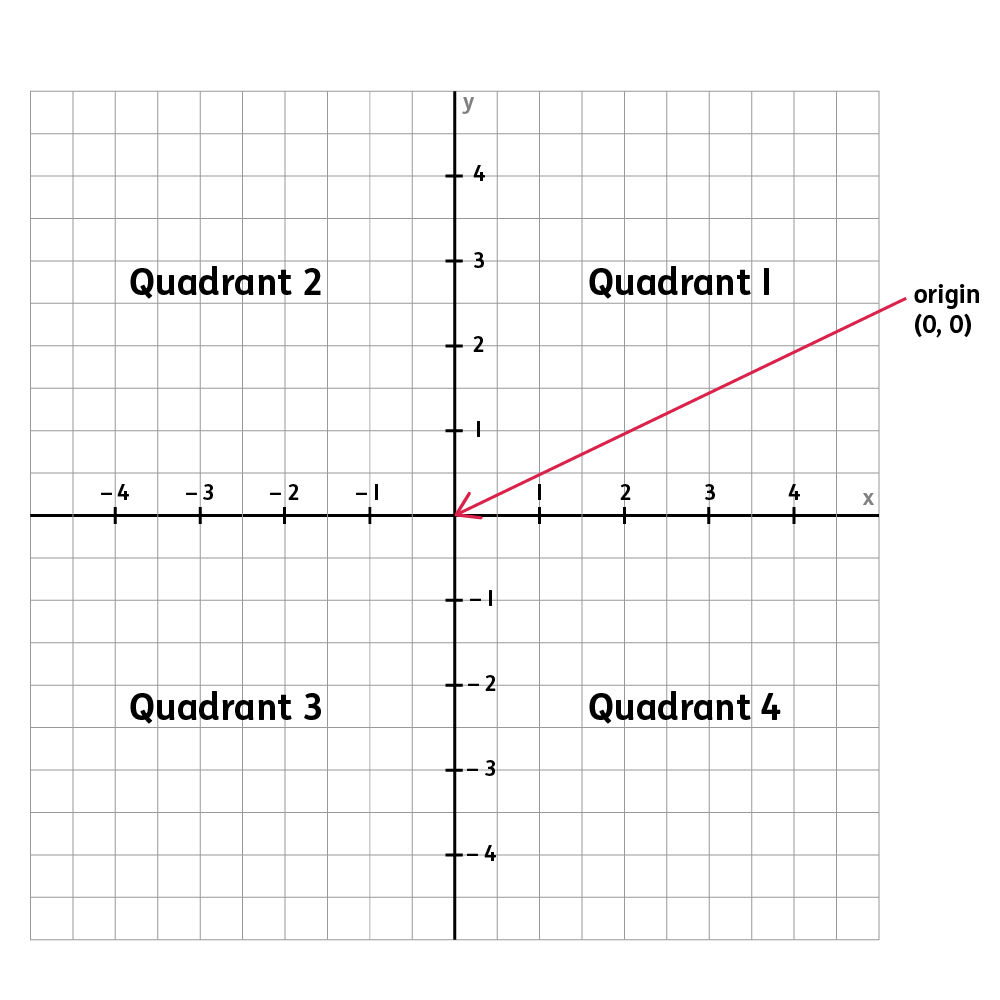Your child will need to be able to describe and record the position of simple shapes using all four quadrants. They will use positive and negative values for x and y and will plot points on a grid to draw a given polygon.

Think of a shape that covers the four quadrants and then give your child the coordinates to see if they can draw it. You could have a go using these coordinates:

A = (2,4)

B = (5,3)

C = (-2,1)

D = (-4,4)

E = (-6,-3)

F = (-2,-4)

G = (2,-3)

H = (5,-2)

Then, see if they can do the same for you!